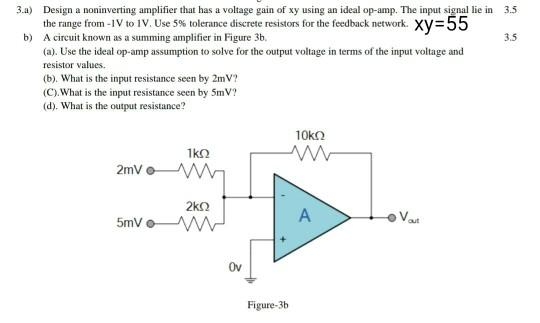# Question Solved1 Answer3.5 3.a) Design a noninverting amplifier that has a voltage gain of xy using an ideal op-amp. The input signal lie in 3.5 the range from 1 to IV. Use 5% tolerance discrete resistors for the feedback network. Xy=55 b) A circuit known as a summing amplifier in Figure 3b. (a). Use the ideal op-amp assumption to solve for the output voltage in terms of the input voltage and resistor values. (b). What is the input resistance seen by 2m V? (C). What is the input resistance seen by SmV? (d). What is the output resistance? 10kΩ 1kΩ 2mV 2k2 5mV A Var Ov Figure-3b7NYMVN The Asker · Electrical EngineeringTranscribed Image Text: 3.5 3.a) Design a noninverting amplifier that has a voltage gain of xy using an ideal op-amp. The input signal lie in 3.5 the range from 1 to IV. Use 5% tolerance discrete resistors for the feedback network. Xy=55 b) A circuit known as a summing amplifier in Figure 3b. (a). Use the ideal op-amp assumption to solve for the output voltage in terms of the input voltage and resistor values. (b). What is the input resistance seen by 2m V? (C). What is the input resistance seen by SmV? (d). What is the output resistance? 10kΩ 1kΩ 2mV 2k2 5mV A Var Ov Figure-3b
More
Transcribed Image Text: 3.5 3.a) Design a noninverting amplifier that has a voltage gain of xy using an ideal op-amp. The input signal lie in 3.5 the range from 1 to IV. Use 5% tolerance discrete resistors for the feedback network. Xy=55 b) A circuit known as a summing amplifier in Figure 3b. (a). Use the ideal op-amp assumption to solve for the output voltage in terms of the input voltage and resistor values. (b). What is the input resistance seen by 2m V? (C). What is the input resistance seen by SmV? (d). What is the output resistance? 10kΩ 1kΩ 2mV 2k2 5mV A Var Ov Figure-3b